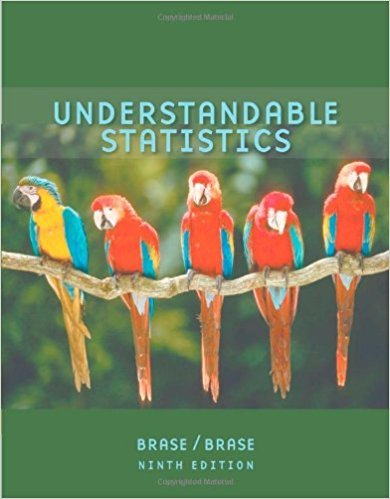×
×

# Solutions for Chapter 9.1: HYPOTHESIS TESTING## Full solutions for Understandable Statistics | 9th Edition

ISBN: 9780618949922Solutions for Chapter 9.1: HYPOTHESIS TESTING

Solutions for Chapter 9.1
4 5 0 403 Reviews
21
1
##### ISBN: 9780618949922

This expansive textbook survival guide covers the following chapters and their solutions. Since 14 problems in chapter 9.1: HYPOTHESIS TESTING have been answered, more than 38336 students have viewed full step-by-step solutions from this chapter. Chapter 9.1: HYPOTHESIS TESTING includes 14 full step-by-step solutions. This textbook survival guide was created for the textbook: Understandable Statistics, edition: 9. Understandable Statistics was written by and is associated to the ISBN: 9780618949922.

Key Statistics Terms and definitions covered in this textbook
• Attribute

A qualitative characteristic of an item or unit, usually arising in quality control. For example, classifying production units as defective or nondefective results in attributes data.

• Bayes’ theorem

An equation for a conditional probability such as PA B ( | ) in terms of the reverse conditional probability PB A ( | ).

• Bimodal distribution.

A distribution with two modes

• Binomial random variable

A discrete random variable that equals the number of successes in a ixed number of Bernoulli trials.

• Combination.

A subset selected without replacement from a set used to determine the number of outcomes in events and sample spaces.

• Components of variance

The individual components of the total variance that are attributable to speciic sources. This usually refers to the individual variance components arising from a random or mixed model analysis of variance.

• Conditional probability distribution

The distribution of a random variable given that the random experiment produces an outcome in an event. The given event might specify values for one or more other random variables

• Continuous random variable.

A random variable with an interval (either inite or ininite) of real numbers for its range.

• Correlation matrix

A square matrix that contains the correlations among a set of random variables, say, XX X 1 2 k , ,…, . The main diagonal elements of the matrix are unity and the off-diagonal elements rij are the correlations between Xi and Xj .

• Covariance matrix

A square matrix that contains the variances and covariances among a set of random variables, say, X1 , X X 2 k , , … . The main diagonal elements of the matrix are the variances of the random variables and the off-diagonal elements are the covariances between Xi and Xj . Also called the variance-covariance matrix. When the random variables are standardized to have unit variances, the covariance matrix becomes the correlation matrix.

• Cumulative sum control chart (CUSUM)

A control chart in which the point plotted at time t is the sum of the measured deviations from target for all statistics up to time t

• Curvilinear regression

An expression sometimes used for nonlinear regression models or polynomial regression models.

• Discrete uniform random variable

A discrete random variable with a inite range and constant probability mass function.

• Distribution free method(s)

Any method of inference (hypothesis testing or conidence interval construction) that does not depend on the form of the underlying distribution of the observations. Sometimes called nonparametric method(s).

• Eficiency

A concept in parameter estimation that uses the variances of different estimators; essentially, an estimator is more eficient than another estimator if it has smaller variance. When estimators are biased, the concept requires modiication.

• Error propagation

An analysis of how the variance of the random variable that represents that output of a system depends on the variances of the inputs. A formula exists when the output is a linear function of the inputs and the formula is simpliied if the inputs are assumed to be independent.

• F-test

Any test of signiicance involving the F distribution. The most common F-tests are (1) testing hypotheses about the variances or standard deviations of two independent normal distributions, (2) testing hypotheses about treatment means or variance components in the analysis of variance, and (3) testing signiicance of regression or tests on subsets of parameters in a regression model.

• Fraction defective

In statistical quality control, that portion of a number of units or the output of a process that is defective.

• Generating function

A function that is used to determine properties of the probability distribution of a random variable. See Moment-generating function

• Harmonic mean

The harmonic mean of a set of data values is the reciprocal of the arithmetic mean of the reciprocals of the data values; that is, h n x i n i = ? ? ? ? ? = ? ? 1 1 1 1 g .

×